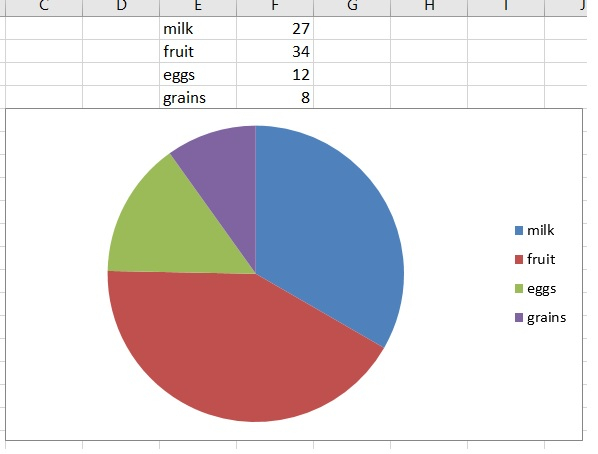# Adding a Chartsheet in an excel sheet using Python XlsxWriter module

In addition to python’s own libraries, there are many external libraries created by individual authors which do a great job of creating additional features in python. Xlsx library is one such library which not only creates excel files containing data from python programs but also creates charts.

## Creating Pie Chart

In the below example we will create a pie chart using the xlsxwriter writer. Here we first define a workbook then add a worksheet to it in the next step we define the data and decide on the columns where the data will be stored in an excel file based on those columns we define a pie chart and again are the chat to a specific location in the worksheet.

## Example

import xlsxwriter

workbook = xlsxwriter.Workbook('pie_chart_example.xlsx')

# Add the data to be plotted.
data = [
['milk', 'fruit', 'eggs', 'grains'],
[27,34,12,8]
]
worksheet.write_column('A1', data)
worksheet.write_column('B1', data)

# Create a new chart object.

# Add a series to the chart.
'categories': '=Sheet1!$A$1:$A$4',
'values': '=Sheet1!$B$1:$B$4'
})

# Insert the chart into the worksheet at a specific position
worksheet.insert_chart('C5', chart)

workbook.close()

Running the above code gives us the following result